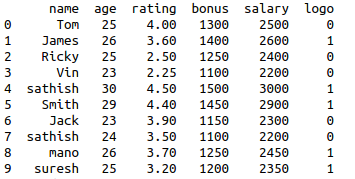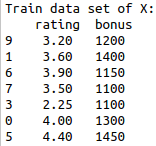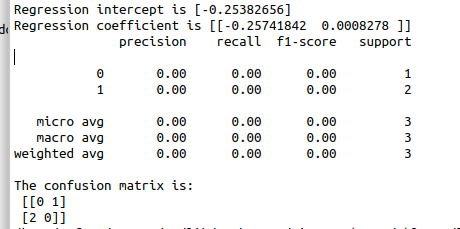• #5, First Floor, 4th Street Dr. Subbarayan Nagar Kodambakkam, Chennai-600 024 Landmark : Samiyar Madam
• pro@slogix.in
• +91- 81240 01111

#### Social List

##### How to build logistic regression model using sklearn in python?
###### Description

To build a logistic regression model for given data set using python.

###### Process

Import libraries.

Define X and y variables.

Build the logistic regression model using sklearn.

Split the sample data into training and test data.

Fit the training data into the regression model.

###### Sapmle Code

#import libraries

import pandas as pd

from sklearn.model_selection import train_test_split

from sklearn.linear_model import LogisticRegression

from sklearn import metrics

from sklearn.metrics import classification_report

Sathish/Pythonfiles/Employee.csv’)

#creating data frame

df=pd.DataFrame(data)

print(df)

#assigning the independent variable

X = df[[‘rating’,’bonus’]]

#assigning the dependent variable

y = df[‘logo’]

#split data in training and test data

X_train, X_test, y_train, y_test = train_test_split(X, y, test_size=0.3, random_state=0)

print(“Train data set of X:\n”,X_train)

print(“Train data set of y:\n”,y_train)

#build the model

logmodel = LogisticRegression()

logmodel.fit(X_train,y_train)

#predictions

predictions = logmodel.predict(X_test)

#regression co-efficients and intercept

print(“Regression intercept is”,logmodel.intercept_)

print(“Regression coefficient is”,logmodel.coef_)

#classification report

print(classification_report

(y_test,predictions))

cm = metrics.confusion_matrix(y_test, predictions)

print(“The confusion matrix is:\n”,cm)

#####Take more sample for more precision and recall values.

###### Screenshots